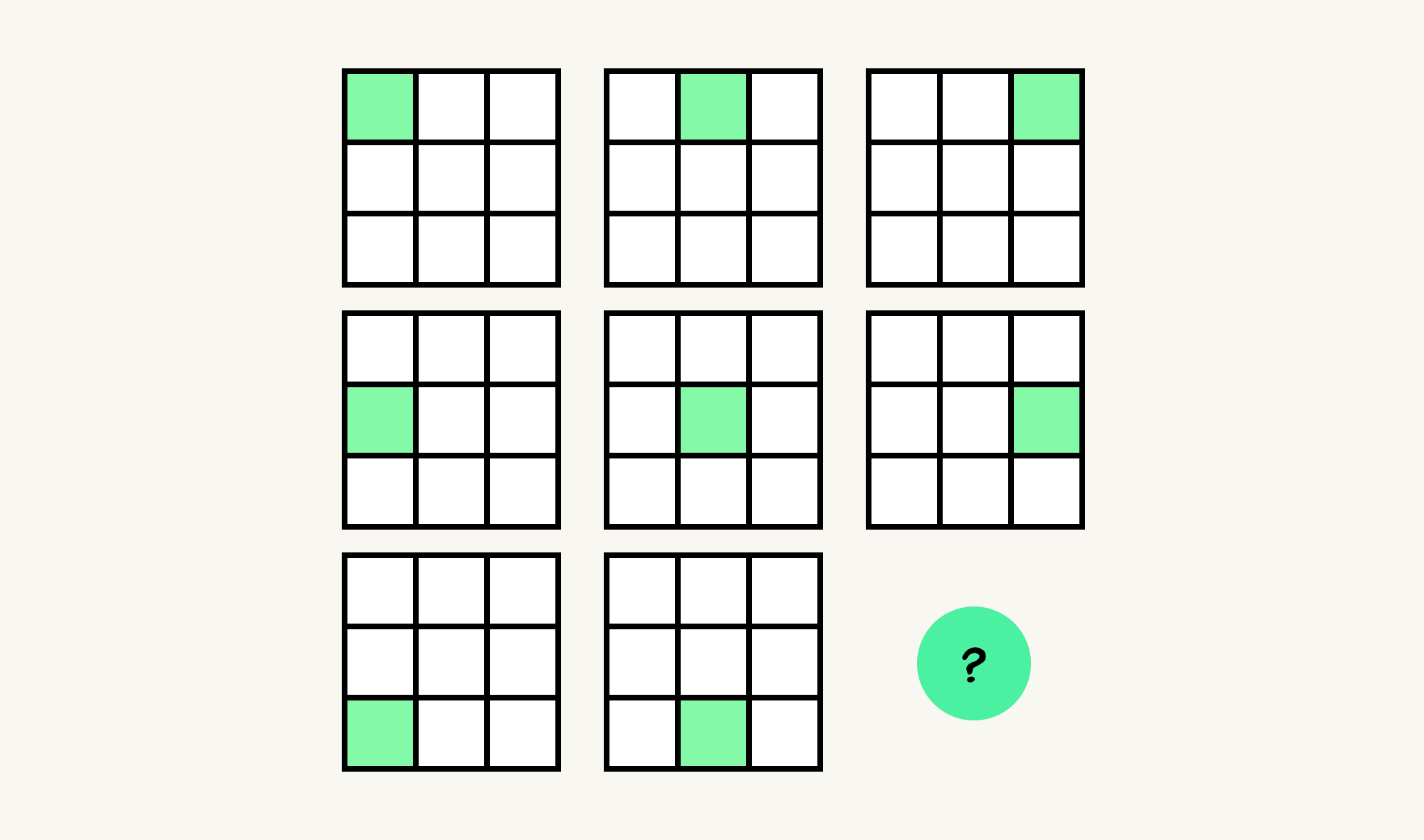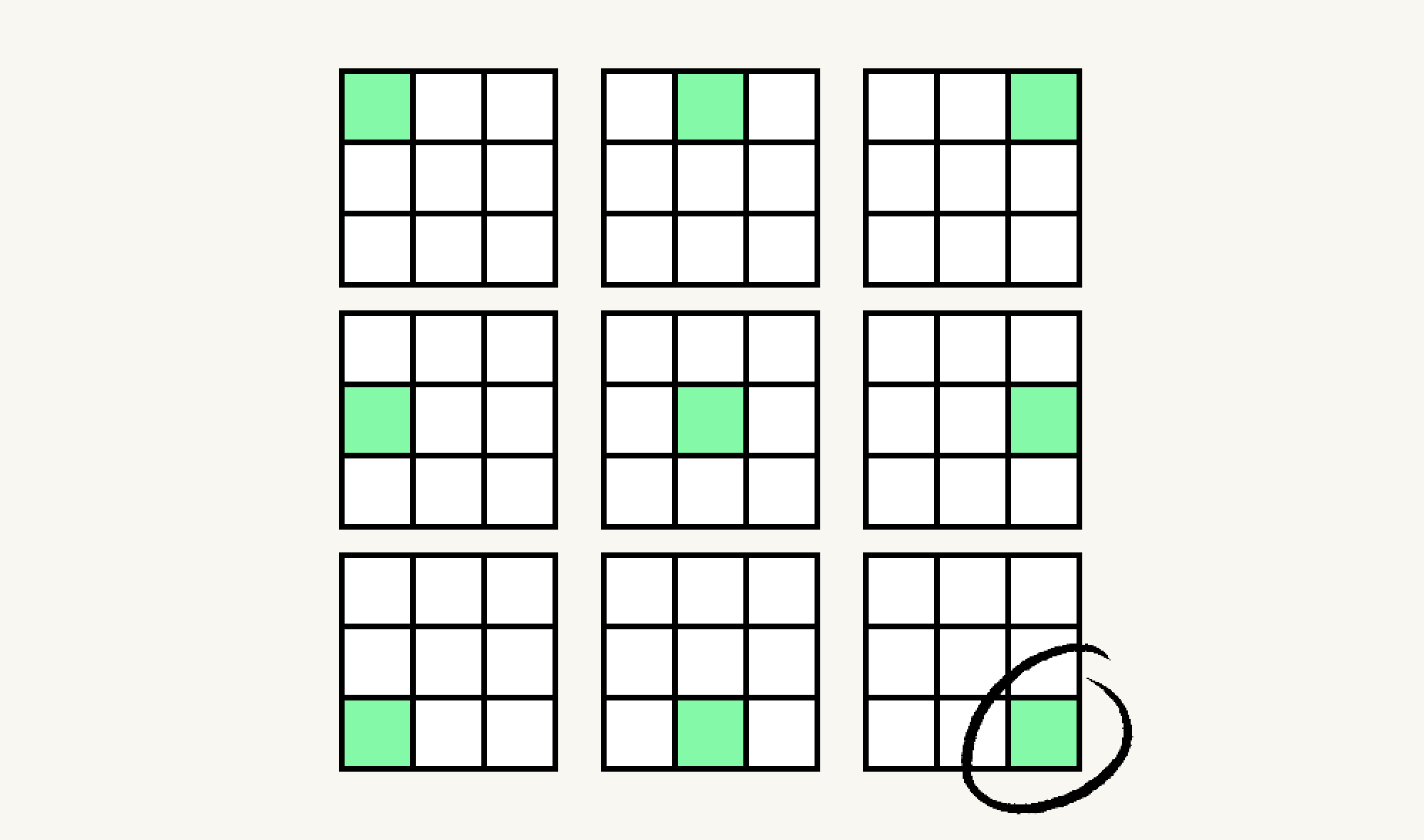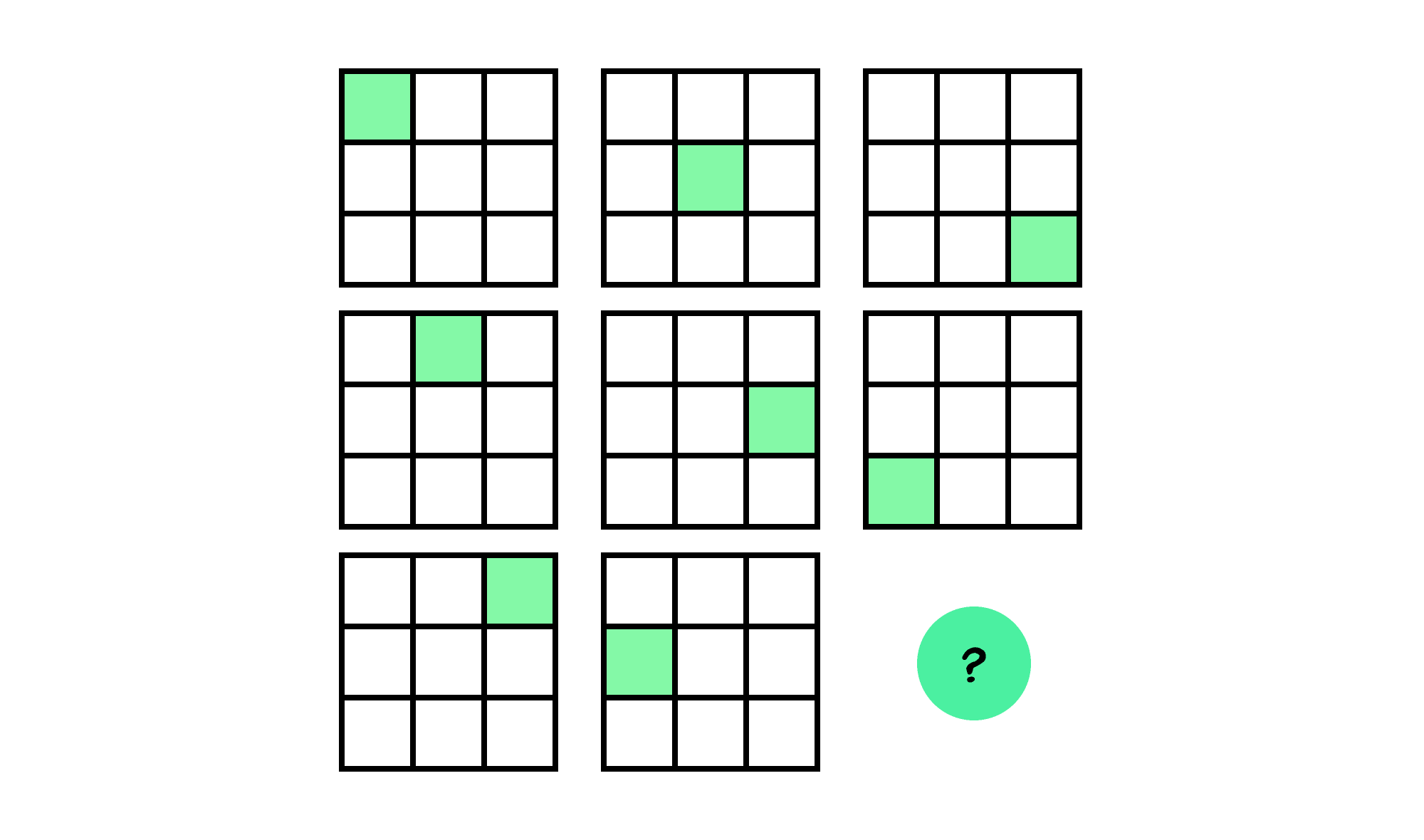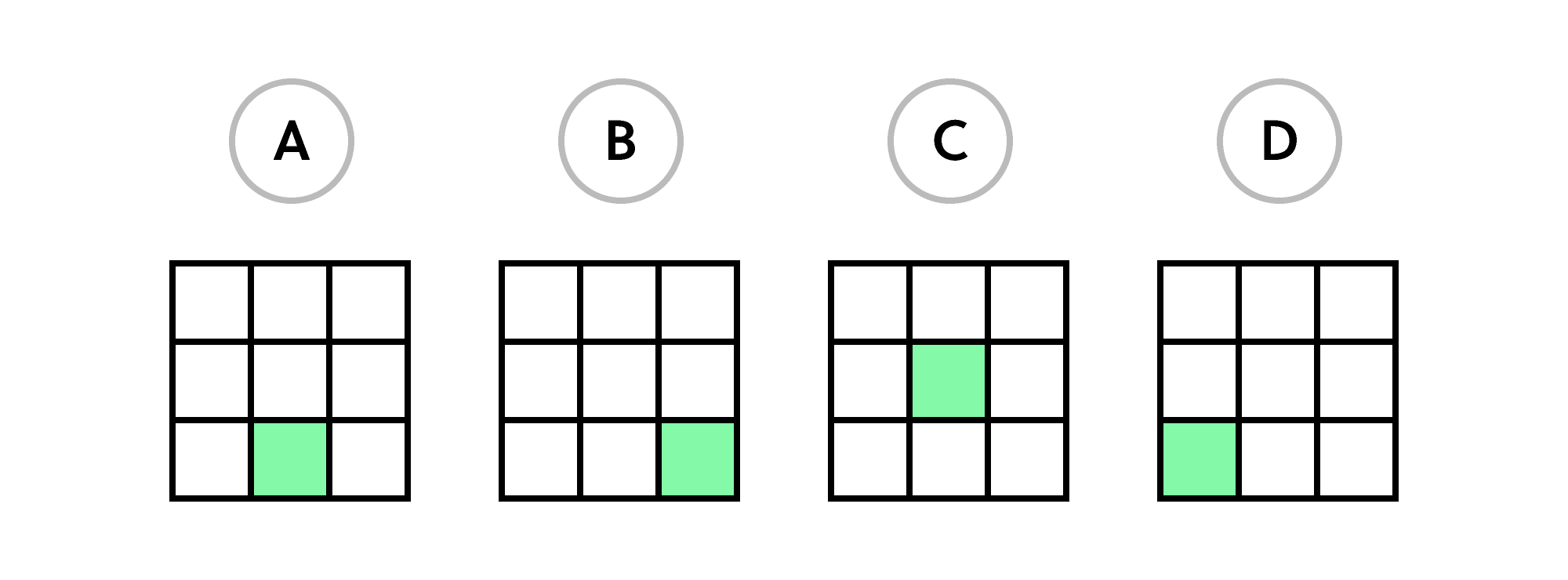Back

## Find the Pattern

When attempting to describe a pattern, one approach is to try to find a rule that describes what is happening. If the rule can be applied in every case available to you, it's a valid way to decode the pattern.

Keep reading to see an example, or try the pattern below for yourself.What rule describes the pattern above?

There might be many different ways to put what's happening here into words. One way to describe it, though, is this: in the figure above, every $3\times 3$ grid is shaded in the square that corresponds to the position of the $3\times 3$ grid among nine of them.

Two examples: the $3\times 3$ grid in the center has its central square shaded, while the $3\times 3$ grid in the upper left has its small upper left square shaded.

Given this, we'd expect the bottom right square to be shaded in the bottom right, and that's the most reasonable way to complete this particular pattern.What's the most reasonable way to complete the pattern below?

# Today's ChallengeWhich of these is the best fit to complete the pattern shown here?×